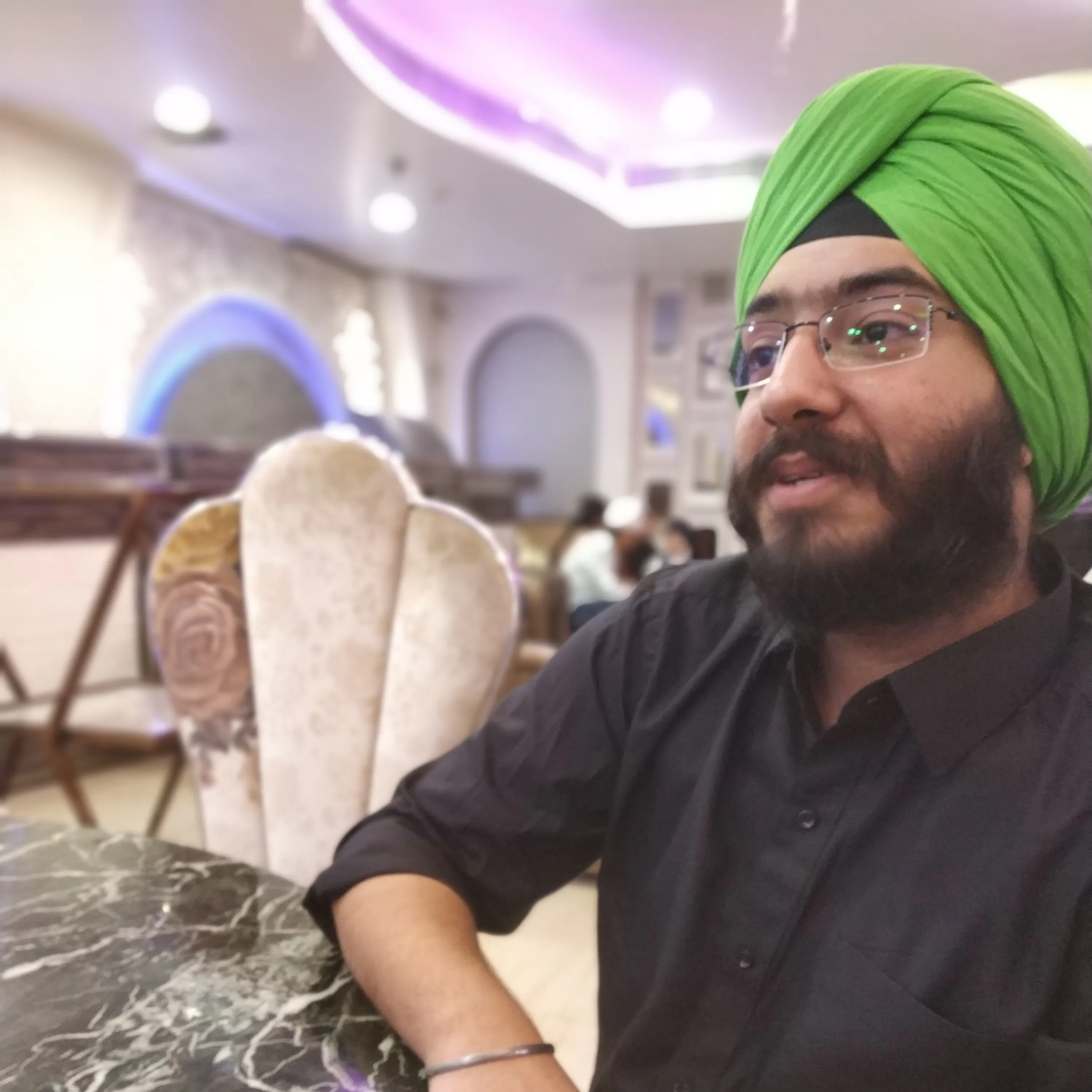# Real Harry Potter Wand with Computer Vision

This project shows how you can bring the Wizarding of Harry Potter to reality with computer vision and machine learning!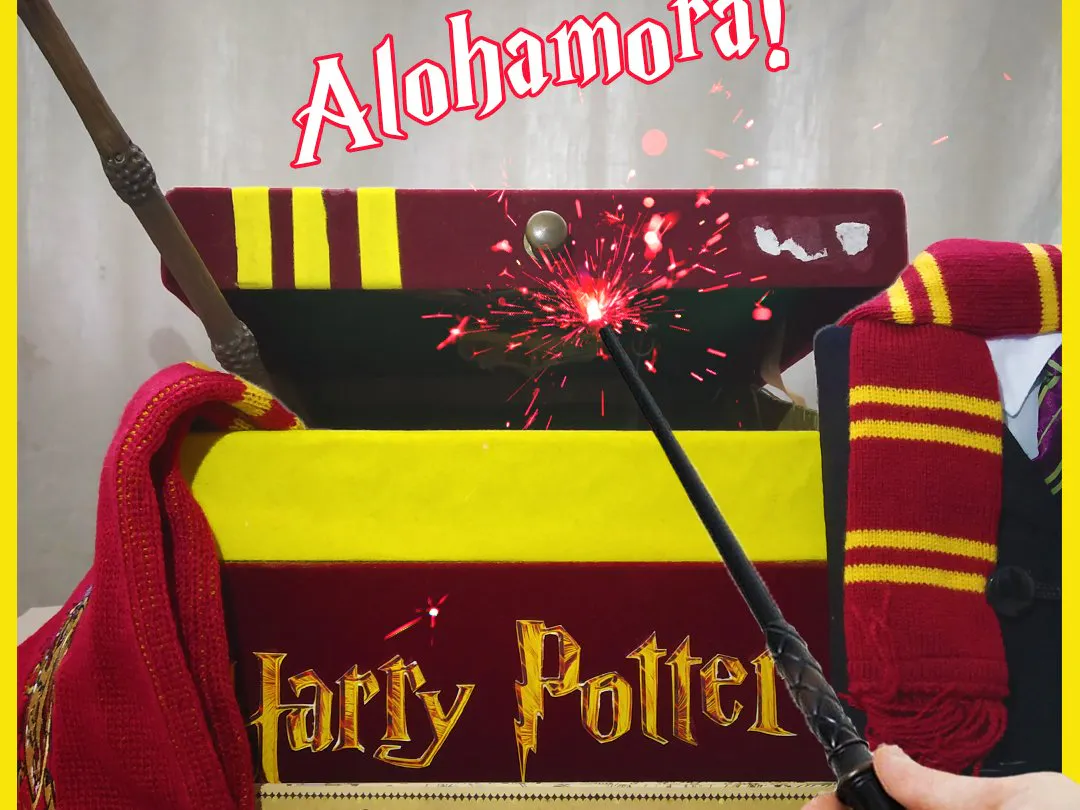## Things used in this project

### Hardware componentsRaspberry Pi 3 Model B
×1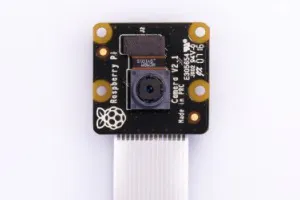Raspberry Pi Pi NoIR Camera V2
×1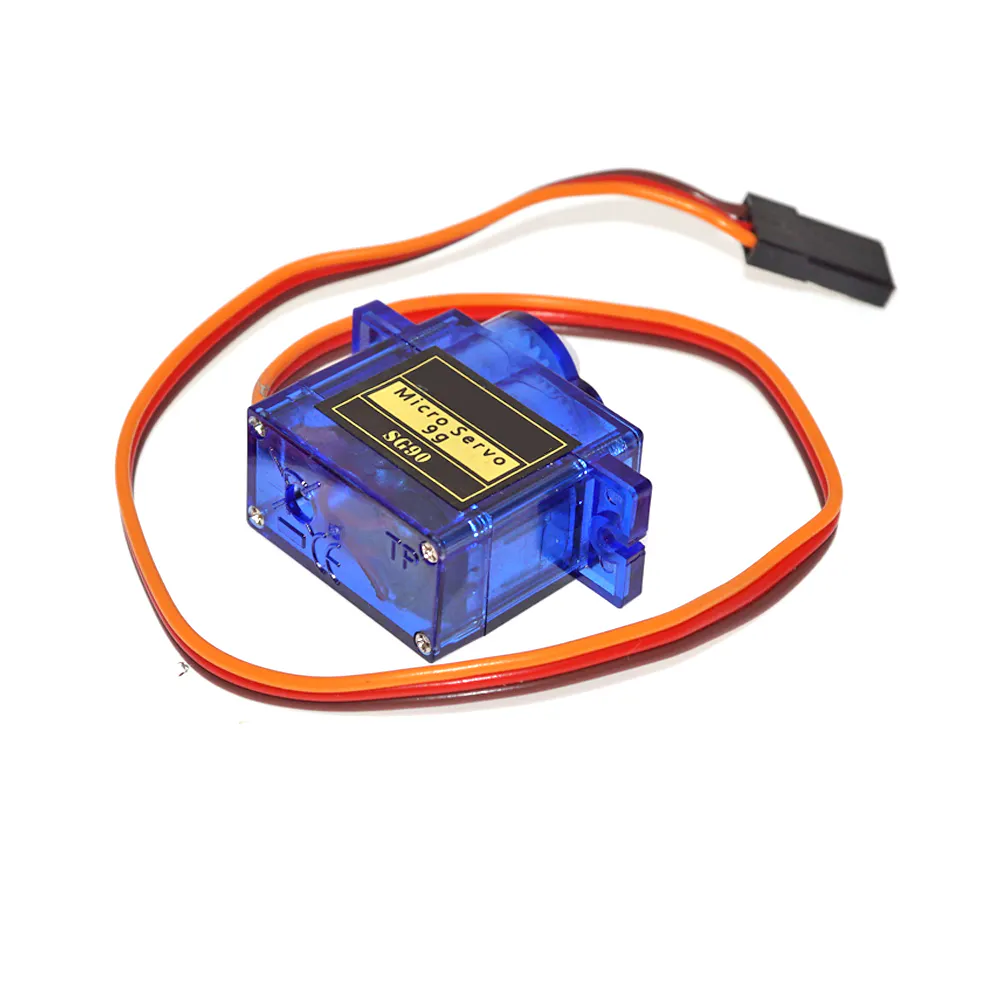SG90 Micro-servo motor
×1Jumper wires (generic)
×1
×1
 infrared leds
×10
 Harry Potter Wand from Wizarding World of Harry Potter at Universal Studios
×1

### Software apps and online services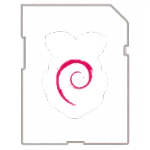Raspberry Pi Raspbian

### Hand tools and fabrication machines3D Printer (generic)Hot glue gun (generic)Soldering iron (generic)

## Custom parts and enclosures

### Night Vision Camera Enclosure

This is a .skp file which is the design of a Camera enclosure for the NoIR(No infrared) raspberry pi camera module. This design was created using sketchup and printed with black filament in about 40 min

## Schematics

### Infrared Leds For Camera Enclosure

This schematic shows how the 10 infrared leds are to be connected on the Night Vision Cammera mount after printing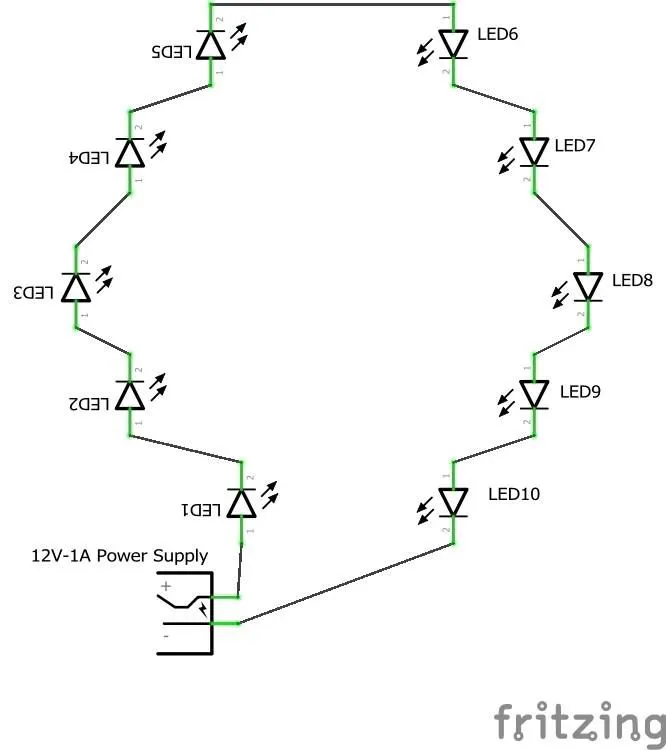## Code

### Training_SVM

Python
This is the python code which uses the scikit-learn module to create a SVM classifier and create a trained model for Handwritten English Alphabet Recognition.
This program imports the the downloaded dataset and slices it to get dataset of 2 letters, A and C, and then trains a SVM classifier, prints its accuracy and saves the trained model as alphabet_classifier.pkl
```import pandas as pd
from pandas import DataFrame
from sklearn.externals import joblib
from sklearn.svm import SVC
from sklearn.model_selection import train_test_split
from sklearn.metrics import accuracy_score

print("....Reading DataSet and Creating Pandas DataFrame....")
alphabet_data = pd.read_csv("") # enter path of the saved .csv dataset file
print("...DataFrame Created...")

print("...Slicing and creating initial training and testing set...")
# Dataset of letter A containg features
X_Train_A = alphabet_data.iloc[:13869, 1:]
# Dataset of letter A containg labels
Y_Train_A = alphabet_data.iloc[:13869, 0]
# Dataset of letter C containg features
X_Train_C = alphabet_data.iloc[22537:45946, 1:]
# Dataset of letter C containg labels
Y_Train_C = alphabet_data.iloc[22537:45946, 0]
# Joining the Datasets of both letters
X_Train = pd.concat([X_Train_A, X_Train_C], ignore_index=True)
Y_Train = pd.concat([Y_Train_A, Y_Train_C], ignore_index=True)
print("...X_Train and Y_Train created...")

train_features, test_features, train_labels, test_labels = train_test_split(X_Train, Y_Train, test_size=0.25, random_state=0)

# SVM classifier created
clf = SVC(kernel='linear')
print("")
print("...Training the Model...")
clf.fit(train_features, train_labels)
print("...Model Trained...")

labels_predicted = clf.predict(test_features)
print(test_labels)
print(labels_predicted)
accuracy = accuracy_score(test_labels, labels_predicted)

print("")
print("Accuracy of the model is:  ")
print(accuracy)

print("...Saving the trained model...")
joblib.dump(clf, "alphabet_classifier.xml", compress=3)
print("...Model Saved...")
```

### HarryPotterWandcv

Python
After creating the trained model, the final step is to write a python program which is does all the work related to video and image processing.

This program gets the last frame from the real-time video with the complete character drawn on it. Performs some pre-processing to make it fit for prediction and then saves it.
Using the subprocess module, this code runs the HarryPotterWandsklearn.py file(For making prediction) and gets its output according to which it controls the servo attached to pin 12 of the Raspberry Pi.
```# For camera module
from picamera import PiCamera
from picamera.array import PiRGBArray

# For servo control
import RPi.GPIO as GPIO

# For image processing
import numpy as np
import cv2

import time
import subprocess

# initializing Picamera
camera = PiCamera()
camera.framerate = 33
camera.resolution = (640, 480)
rawCapture = PiRGBArray(camera, size = (640, 480))

# setting up pin 12 for servo as PWM
GPIO.setmode(GPIO.BOARD)
GPIO.setup(12, GPIO.OUT)
servo = GPIO.PWM(12, 50)
servo.start(0)

# Define parameters for the required blob
params = cv2.SimpleBlobDetector_Params()

# setting the thresholds
params.minThreshold = 150
params.maxThreshold = 250

# filter by color
params.filterByColor = 1
params.blobColor = 255

# filter by circularity
params.filterByCircularity = 1
params.minCircularity = 0.68

# filter by area
params.filterByArea = 1
params.minArea = 30
# params.maxArea = 1500

# creating object for SimpleBlobDetector
detector = cv2.SimpleBlobDetector_create(params)

flag = 0
points = []
lower_blue = np.array([255, 255, 0])
upper_blue = np.array([255, 255, 0])

# Function for Pre-processing
def last_frame(img):
cv2.imwrite("/home/pi/Desktop/lastframe1.jpg", img)
img = cv2.GaussianBlur(img, (5, 5), 0)
cv2.imwrite("/home/pi/Desktop/lastframe2.jpg", img)
retval, img = cv2.threshold(img, 80, 255, cv2.THRESH_BINARY)
cv2.imwrite("/home/pi/Desktop/lastframe3.jpg", img)
img = cv2.resize(img, (28, 28), interpolation=cv2.INTER_AREA)
cv2.imwrite("/home/pi/Desktop/lastframe4.jpg", img)
img = cv2.dilate(img, (3, 3))
cv2.imwrite("/home/pi/Desktop/lastframe.jpg", img)
output = subprocess.check_output(['python3', '/home/pi/Desktop/HarryPotterWandsklearn.py'])
print(output)
if output == "0":
print("Alohamora!!")
servo.ChangeDutyCycle(6.5)
time.sleep(1.5)
print("Box Opened!!")
if output == "2":
print("Close!!")
servo.ChangeDutyCycle(3.5)
print("Box Closed!!")
time.sleep(1.5)

time.sleep(0.1)

for image in camera.capture_continuous(rawCapture, format='bgr', use_video_port=True):
frame = image.array
frame = cv2.resize(frame, (frame.shape//2, frame.shape//2))
frame =cv2.cvtColor(frame, cv2.COLOR_BGR2GRAY)

#detecting keypoints
keypoints = detector.detect(frame)
frame_with_keypoints = cv2.drawKeypoints(frame, keypoints, np.array([]), (0, 0, 255), cv2.DRAW_MATCHES_FLAGS_DRAW_RICH_KEYPOINTS)

#starting and ending circle
frame_with_keypoints = cv2.circle(frame_with_keypoints, (140, 70), 6, (0, 255, 0), 2)
frame_with_keypoints = cv2.circle(frame_with_keypoints, (190, 140), 6, (0, 0, 255), 2)

#points_array = cv2.KeyPoint_convert(keypoints)
points_array = cv2.KeyPoint_convert(keypoints)

if flag == 1:
# Get coordinates of the center of blob from keypoints and append them in points list
points.append(points_array)

# Draw the path by drawing lines between 2 consecutive points in points list
for i in range(1, len(points)):
cv2.line(frame_with_keypoints, tuple(points[i-1]), tuple(points[i]), (255, 255, 0), 3)

if len(points_array) != 0:

if flag == 1:
if int(points_array) in range(185, 195) and int(points_array) in range(135, 145):
print("Tracing Done!!")
frame_with_keypoints = cv2.inRange(frame_with_keypoints, lower_blue, upper_blue)
last_frame(frame_with_keypoints)
break

if flag == 0:
if int(points_array) in range(135, 145) and int(points_array) in range(65, 75):
time.sleep(0.5)
print("Start Tracing!!")
flag = 1

cv2.imshow("video",frame_with_keypoints)
cv2.imshow("video 2",frame)
rawCapture.truncate(0)
key = cv2.waitKey(1) & 0xFF
if key == ord('q'):
break

cv2.destroyAllWindows()
servo.stop()
GPIO.cleanup()
```

### HarryPotterWandsklearn

Python
This code file is accessed from the HarryPotterWandcv.py file using the subprocess module. It loads the processed last frame using the pillow module and makes the prediction by loading the saved SVM classifier and prints the prediction.
```from PIL import Image
from sklearn.externals import joblib
import numpy as np

img = Image.open("/home/pi/Desktop/lastframe.jpg")

# Converting image to numpy array
img = np.array(img)
# Converting the numpy array to 1-Dimensional array
img = img.reshape(1, -1)

prediction = clf.predict(img)
print(prediction)
```

## Credits

### Jasmeet Singh

12 projects • 15 followers